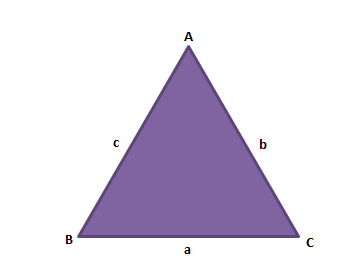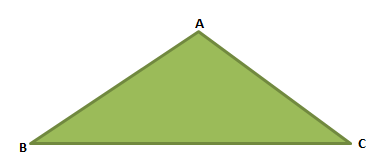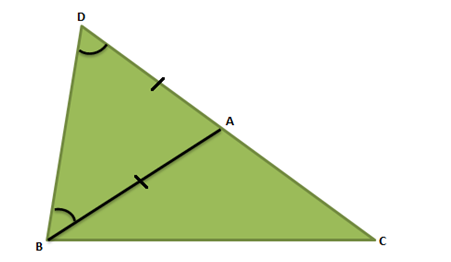# Triangle Inequality Theorem

According to triangle inequality theorem, for any given triangle, the sum of two sides of a triangle is always greater than the third side. A polygon bounded by three line-segments is known as the Triangle. It is the smallest possible polygon. A triangle has three sides, three vertices, and three interior angles. The types of triangles are based on its angle measure and length of the sides. The inequality theorem is applicable for all types triangles such as equilateral, isosceles and scalene. Now let us learn this theorem in details with its proof.

## Triangle Inequality Theorem Proof

The triangle inequality theorem describes the relationship between the three sides of a triangle. According to this theorem, for any triangle, the sum of lengths of two sides is always greater than the third side. In other words, this theorem specifies that the shortest distance between two distinct points is always a straight line.

Consider a ∆ABC as shown below, with a, b and c as the side lengths.The triangle inequality theorem states that:

a < b + c,

b < a + c,

c < a + b

In any triangle, the shortest distance from any vertex to the opposite side is the Perpendicular. In figure below, XP is the shortest line segment from vertex X to side YZ.Let us prove the theorem now for a triangle ABC.Triangle ABC

To Prove: |BC|< |AB| + |AC|

Construction: Consider a ∆ABC. Extend the side AC to a point D such that AD = AB as shown in the fig. below.Proof of triangle inequality theorem

 S.No Statement Reason 1. |CD|= |AC| + |AD| From figure 3 2. |CD|= |AC| + |AB| AB = AD, ∆ADB is an isosceles triangle 3. ∠DBA <∠DBC Since ∠DBC = ∠DBA+∠ABD 4. ∠ADB<∠DBC ∆ADB is an isosceles triangle and ∠ADB = ∠DBA 5. |BC|<|CD| Side opposite to greater angle is larger 6. |BC|<|AC| + |AB| From statements 3 and 4

Thus, we can conclude that the sum of two sides of a triangle is greater than the third side.

## Video Lesson on BPT and Similar Triangles### Example Problems

Q.1. If 4cm, 8cm and 2cm are the measures of three lines segment. Can it be used to draw a triangle?

Solution: The triangle is formed by three line segments 4cm, 8cm and 2cm, then it should satisfy the inequality theorem.

Hence, let us check if the sum of two sides is greater than the third side.

4 + 8 > 2 ⇒ 12 > 2 ⇒ True

8 + 2 > 4 ⇒ 10 > 4 ⇒ True

4 + 2 > 8 ⇒ 6 > 8 ⇒ False

Therefore, the sides of the triangle do not satisfy the inequality theorem. So, we cannot construct a triangle with these three line-segments.

Q.2: Could a triangle have side length as 6cm, 7cm and 5cm?

Solution: If 6cm, 7cm and 5cm are the sides of the triangle, then they should satisfy inequality theorem.

Hence,

6 + 7 > 5 ⇒ 13 > 5 ⇒ True

7 + 5 > 6 ⇒ 12 > 6 ⇒ True

6 + 5 > 7 ⇒ 11 > 7 ⇒ True

All the three conditions are satisfied, therefore a triangle could have side length as 6cm, 7cm and 5cm

Q.3: If the two sides of a triangle are 2 and 7. Find all the possible lengths of the third side.

Solution: To find the possible values of the third side of the triangle we can use the formula:

A difference of two sides< Unknown side < Sum of the two sides

7 -2 < x < 7 + 2

5 < x <9

There could be any value for the third side between 5 and 9.